# 5.4 Equilibrium Solutions and Phase Portraits 1. 2 3 3 2 . (a) Draw direction field....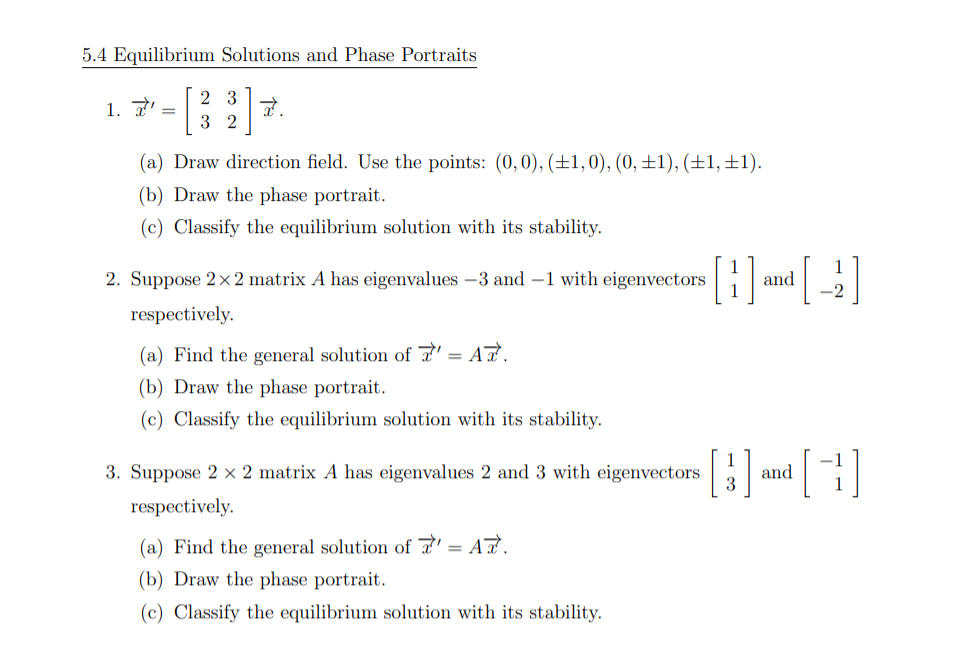5.4 Equilibrium Solutions and Phase Portraits 1. 2 3 3 2 . (a) Draw direction field. Use the points: (0,0), (+1,0), (0, +1), (+1, +1). (b) Draw the phase portrait. (c) Classify the equilibrium solution with its stability. 11 and 2. Suppose 2 x 2 matrix A has eigenvalues – 3 and -1 with eigenvectors respectively. (a) Find the general solution of 7' = A. (b) Draw the phase portrait. (C) Classify the equilibrium solution with its stability. 3. Suppose 2 x 2 matrix A has eigenvalues 2 and 3 with eigenvectors respectively. [ 3 ] and  (a) Find the general solution of ' = AT. (b) Draw the phase portrait. (c) Classify the equilibrium solution with its stability.

Doubt in any step then comment below.. i will explain you..

By rules and regulations we are allow to do only one problem at a time..so i do only 2nd..

.

please thumbs up for this solution.. thanks..

.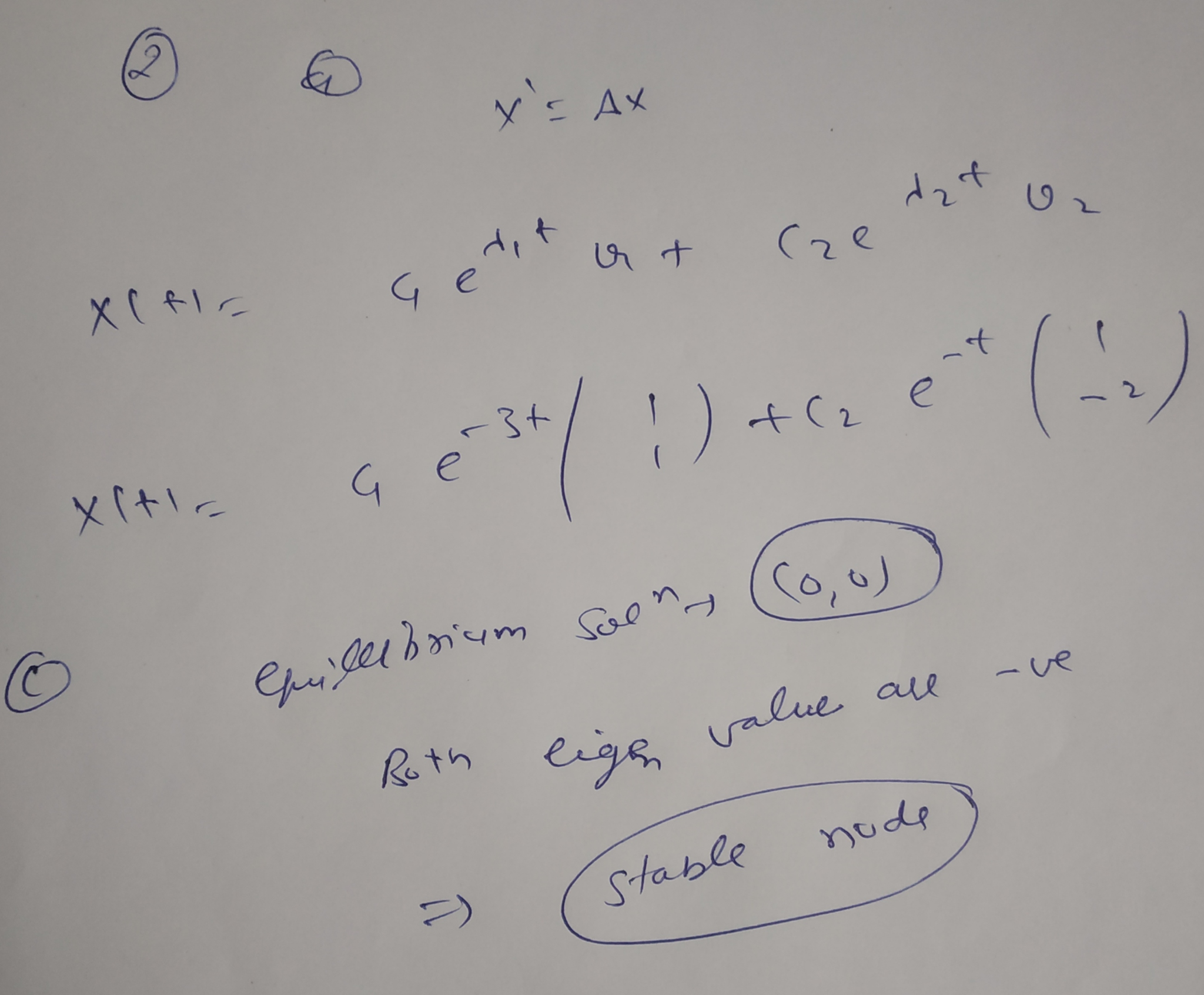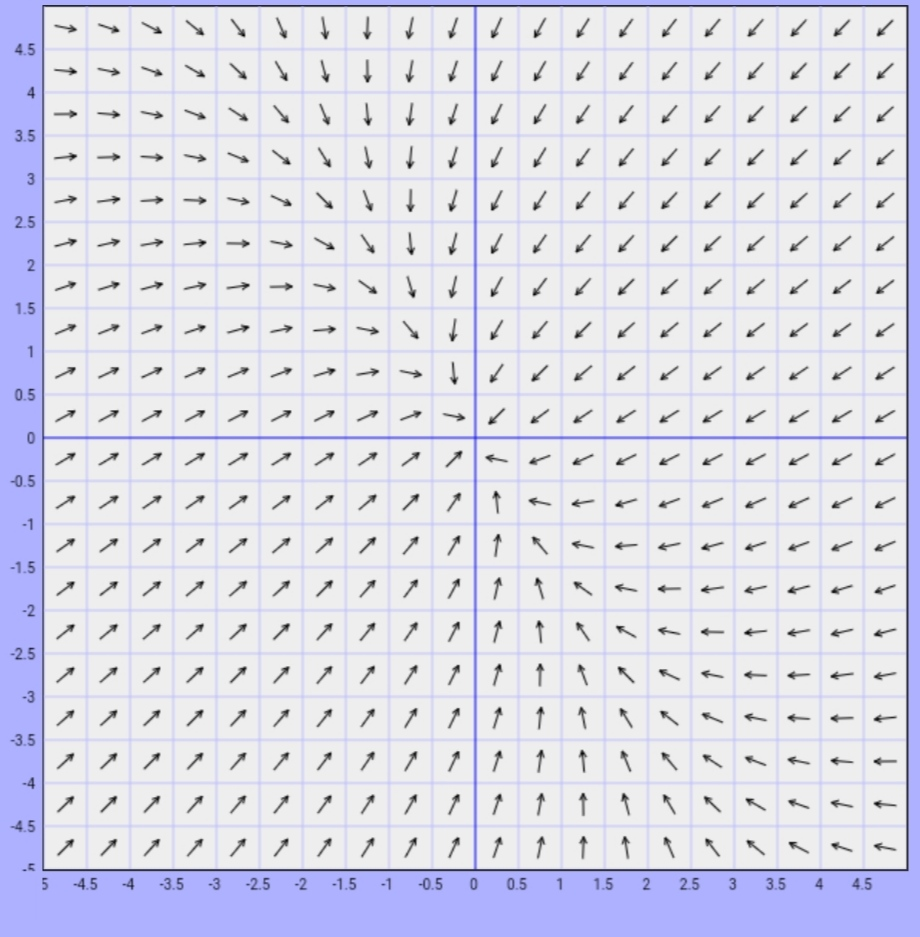##### Add Answer of: 5.4 Equilibrium Solutions and Phase Portraits 1. 2 3 3 2 . (a) Draw direction field....
Similar Homework Help Questions
• ### Suppose 7' = AT, where A is the 2 x 2 matrix below. A= (1 1...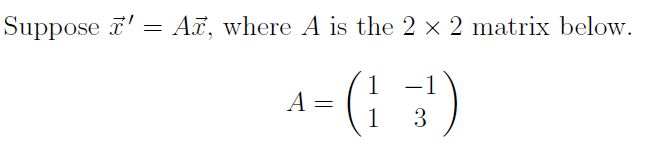Suppose 7' = AT, where A is the 2 x 2 matrix below. A= (1 1 1 3 (a) Determine the eigenvalues and eigenvectors of A. (b) Express the general solution of t' = Az in terms of real valued functions. (c) Sketch the phase portrait of the system. Do not forget to label your axes.

• ### Consider the system of two coupled differential equations: y-cx + dy, x-ax + by, with the equilibrium solution (xe,ye) = (0,0) (a) Rewrite the coupled system as a matrix differential equation and ide...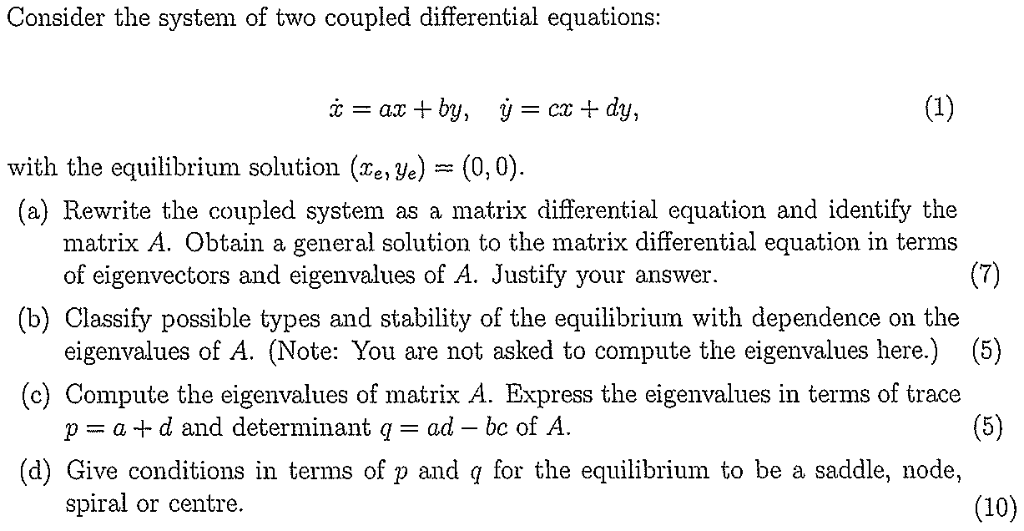Consider the system of two coupled differential equations: y-cx + dy, x-ax + by, with the equilibrium solution (xe,ye) = (0,0) (a) Rewrite the coupled system as a matrix differential equation and identify the matrix A. Obtain a general solution to the matrix differential equation in terms of eigenvectors and eigenvalues of A. Justify your answer (b) Classify possible types and stability of the equilibrium with dependence on the eigenvalues of A. (Note: You are not asked to compute the...

• ### 1) Find the general solution of di = Ay where Then sketch the phase portrait in...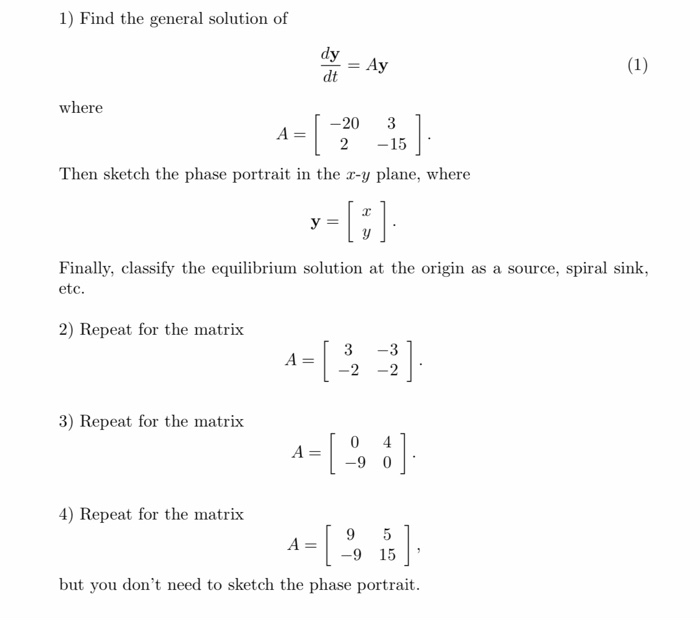1) Find the general solution of di = Ay where Then sketch the phase portrait in the x-y plane, where Finally, classify the equilibrium solution at the origin as a source, spiral sink, etc. 2) Repeat for the matrix | 3 -31 -2 -2] 3) Repeat for the matrix 4 — 4) Repeat for the matrix [95 -9 15 but you don't need to sketch the phase portrait.

• ### Consider the 2-dimensional system of linear equations -2 X' = 2 Note that the coefficient matrix...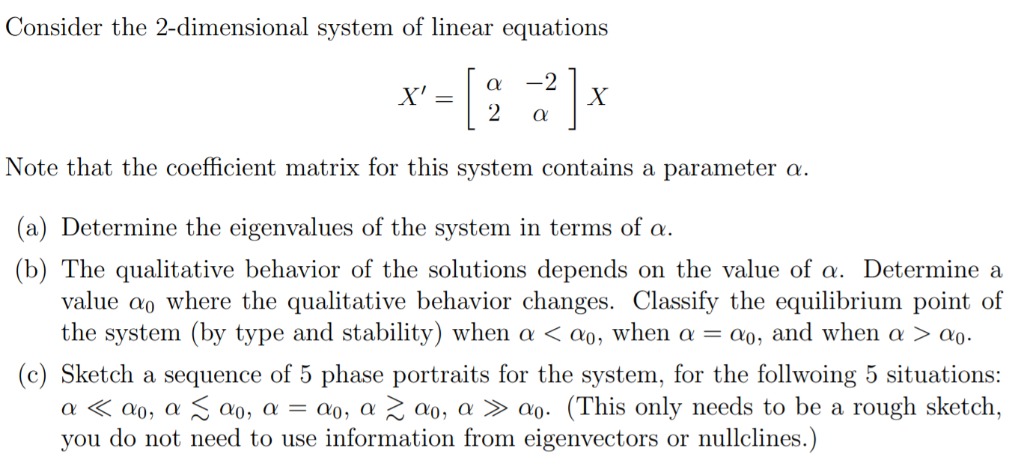Consider the 2-dimensional system of linear equations -2 X' = 2 Note that the coefficient matrix for this system contains a parameter a. (a) Determine the eigenvalues of the system in terms of a (b) The qualitative behavior of the solutions depends value ao where the qualitative behavior changes. Classify the equilibrium point of the system (by type and stability) when a < ao, when a = a), and when a > ao. on the value of a. Determine a...

• ### vector x' = [ the first row is 2 and 8, the second row is -1...

vector x' = [ the first row is 2 and 8, the second row is -1 and -2] vector x (i) Compute the eigenvalues and eigenvectors of the system. (ii) Use the eigenvalues to classify the equilibrium type of the origin. (iii) Use the eigenvectors as guides to plot a phase portrait of the system. (iv) Present a general solution to the system of ODE. (v) Find the particular solution to this system of ODE if vector x(0) = [...

• ### Differential Equations

1. Consider the systemx' = 1 - x,y' = y - x^2(a) (5 Pts.) Find all equilibrium solutions.(b) (5 Pts.) For each equilibrium solution, compute the jacobian matrixand its eigenvalues. Using the the eigenvalues, classify the stability of eachequilibrium solution (e.g. stable spiral, ..)(c) (5 Pts.) Draw the nullclines.(d) (5 Pts.) Indicate, with arrows, the flow of solutions in the (x, y) phase

• ### dx/dt = 4x -x^2 -2xy dy/dt = -y+0.5 xy a) find equilibrium points b) find Jacobian matrix for above system c) find Jacobian matrix at eq. point (0,0) d) draw phase portrait near (0,0) from © e) show...

dx/dt = 4x -x^2 -2xy dy/dt = -y+0.5 xy a) find equilibrium points b) find Jacobian matrix for above system c) find Jacobian matrix at eq. point (0,0) d) draw phase portrait near (0,0) from © e) show at eq. point (4,0) the Jacobian matrix is -4 -8 0 1 f) draw phase portrait near (4,0) from (d) g) at eq. point (2,1) the Jacobian matrix is -2 -4 0.5 0 h) draw phase portrait near (2,1) from (f) i)...

• ### 1 (c) (12 pts) Consider the logistic equation IP 3 Use phase portrait analysis to classify the equilibrium solutions as asymptotically stable, 10 unstable or semi-stable. (ii) Find the general so...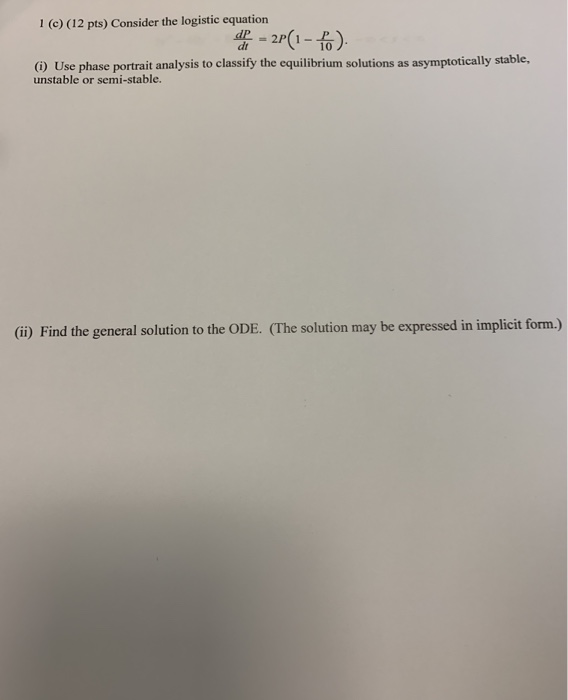1 (c) (12 pts) Consider the logistic equation IP 3 Use phase portrait analysis to classify the equilibrium solutions as asymptotically stable, 10 unstable or semi-stable. (ii) Find the general solution to the ODE. (The solution may be expressed in implicit form.) 1 (c) (12 pts) Consider the logistic equation IP 3 Use phase portrait analysis to classify the equilibrium solutions as asymptotically stable, 10 unstable or semi-stable. (ii) Find the general solution to the ODE. (The solution may be...

• ### 1. (20 marks) This question is about the system of differential equations dY (3 1 (a) Consider the case k 0 i. Dete...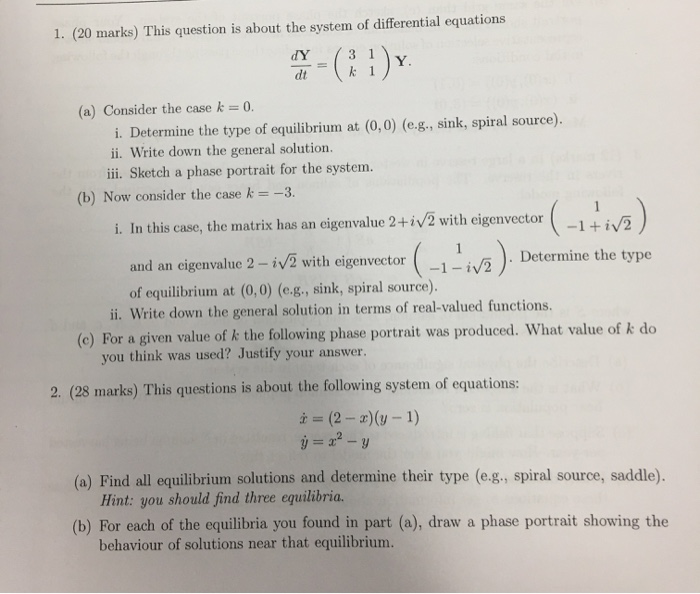1. (20 marks) This question is about the system of differential equations dY (3 1 (a) Consider the case k 0 i. Determine the type of equilibrium at (0,0) (e.g., sink, spiral source). i. Write down the general solution. ili Sketch a phase portrait for the system. (b) Now consider the case k -3. (-1+iv ) i. In this case, the matrix has an eigenvalue 2+i/2 with eigenvector and an eigenvalue 2-W2 with eigenvector Determine the type of equilibrium at...

• ### 1. (This is problem 5 from the second assignment sheet, reprinted here.) Consider the nonlinear system a. Sketch the ulllines and indicate in your sketch the direction of the vector field in each...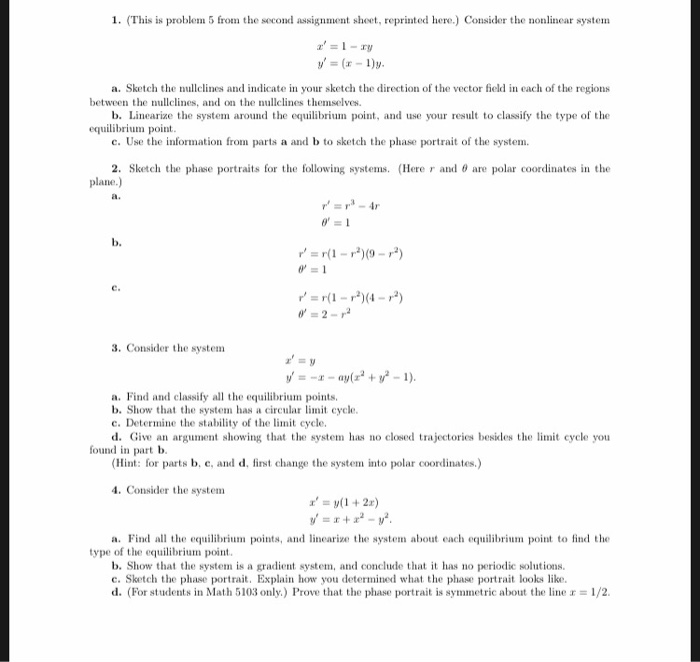1. (This is problem 5 from the second assignment sheet, reprinted here.) Consider the nonlinear system a. Sketch the ulllines and indicate in your sketch the direction of the vector field in each of the regions b. Linearize the system around the equilibrium point, and use your result to classify the type of the c. Use the information from parts a and b to sketch the phase portrait of the system. 2. Sketch the phase portraits for the following systems...

Free Homework App